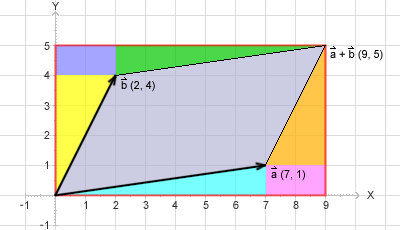﻿ blueling.untergrund.net

# Area of a Paralellogram

The area of a paralellogram is one of the most elementary formulars in computational geometry.
Here are some examples of its application:
• Calculating the area of a triangle or an arbitrary (convex and concave) polygon
• Test if a point lies left, right or on a line (test if three points are ordered clockwise (CW) or counter clockwise (CCW))
• Test if a polygon is convex or concave
• Test if a point lies inside a cone given by three points
• Test if two lines intersect without calculating the intersection point
• Sorting points by "leftness" as a preparing step for Graham's convex hull algorithm
The area of a paralellogram spanned by two vectors a and b can be expressed in R2 by the 2 x 2 determinant:
 A(P) = ax  ay bx  by = ax * by - ay * bx

It turns out that this can easily be shown geometrically:
(For simplicity we will assume that all coordinates are positive. I leave it as an exercise for the reader to show that the equation holds for arbitrary cooridnates.)

We will prove the area calculation by a disjoint decomposition of the bounding box around a paralellogram:As it can be easily seen the area of the parallelogram is what remains if we substract from the red bouding-box the four triangles (yellow, green, orange, light turquoise) and the two rectangles in the upper-left and lower-right corner.

Viewed mathematically we have got:

1. area of red bouding box = (ax + bx) * (ay + by)
2. green and light tourquise triangle added together form the rectangle: ax * ay
3. yellow and orange triangle added together form the rectangle: bx * by
4. upper-left and lower rigth rectangle are each a rectangle of bx * ay, thus togher: 2 * ay * bx

Now we put all pieces into a formula and reduce:

A(P) = (ax + bx) * (ay + by) - (ax * ay + bx * by + 2 * ay * bx )
= ax * ay + ax * by + ay * bx + bx * by - ax * ay - bx * by - 2 * ay * bx
= ax * ay + ax * by + ay * bx + bx * by - ax * ay - bx * by - 2 * ay * bx
= ax * by - ay * bx